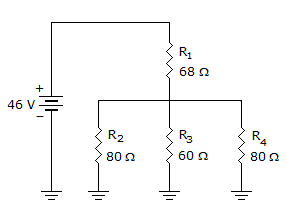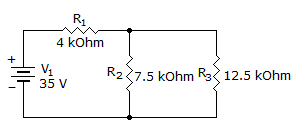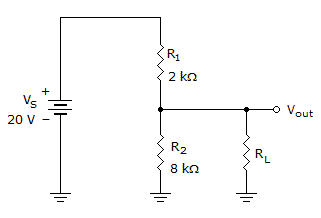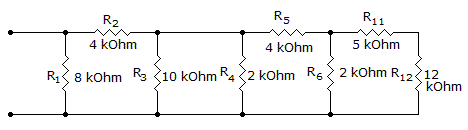# Electronics - Series-Parallel Circuits

### Exercise :: Series-Parallel Circuits - General Questions

11.What is the total resistance of the given circuit?

 A. 92B. 288C. 17.7D. 128Explanation:

No answer description available for this question. Let us discuss.

12.

What are the branch currents I2 and I3?A. I2 = 4 mA, I3 = 2 mA B. I2 = 4.5 mA, I3 = 2.5 mA C. I2 = 2.5 mA, I3 = 1.5 mA D. I2 = 5.5 mA, I3 = 3.5 mA

Explanation:

No answer description available for this question. Let us discuss.

13.If the load in the given circuit is open, what is the unloaded output voltage?

 A. 0 V B. 4 V C. 16 V D. 20 V

Explanation:

No answer description available for this question. Let us discuss.

14.

What is the total resistance?A. 2.2 kB. 3.2 kC. 4.2 kD. 5.2 kExplanation:

No answer description available for this question. Let us discuss.

15.How much voltage is dropped across R3 in the given circuit?

 A. 46 V B. 34 V C. 23 V D. 12 V